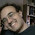sábado, 22 de setembro de 2018

Escudo do Botafogo aparece na prova da Escola Naval

A prova de matemática do concurso de admissão para a Escola Naval, da Marinha do Brasil, realizada no último sábado, 15, teve uma questão interessante, envolvendo o escudo do Botafogo. E aí, você consegue resolver?

(The math test in the admission contest for the Escola Naval, of Brazilian Navy, held on last Saturday, the 15th, had an interesting question, involving the Botafogo club's emblem. So, can you solve it?)

(The pentagram is a star-shaped polygon with 5 vertices, which can be equally distributed in a circle (forming 5 congruent arcs). The pentagram, through its segments, determines 6 internal regions, 5 triangles and 1 pentagon. The pentagon is considered a "neighbor" of all triangles, and there are no mutually neighbor triangles. Thus, using up to 6 distinct colors (black, white, gray, green, yellow and blue), in how many ways can these regions be colored, so that there aren't two neighbor regions with equal colors?)

PCFilho

3 comentários:

1.I'm going to assume that rotations of a particular color scheme are counted as the same, but what about the reflection of a particular color scheme? Is it counted as the same or a distinct color scheme?

1.Rotations are counted as the same. Reflections are not.

2.All right. Here I go, taking a stab at it...

Given info:
You can use up to six different colors to color the pentagram.
Adjacent regions can't be the same color.
The pentagon is adjacent to every triangle, and none of the triangles is adjacent to another triangle.
A rotation of a particular color scheme is treated as the same color scheme.

Question: How many distinct color schemes are possible with this given info?

Case 1: All six colors used.
The first region we color can be any of the six colors, then the second region we color has five possibilities, and so on, until the last region colored must be colored with the last remaining color. So, for this, there seem to be 6*5*4*3*2*1=720 color schemes, but since rotations are counted as the same, we actually have one-fifth this many, or 144 (=720/5) different color schemes.

Case 2: Five colors used.
Two regions must be the same color. The pentagon is adjacent to all of the triangles, so it can't be one of the two same-colored regions. Therefore, we must use one of the colors to color two of the triangles. We can do this in only two ways (either the same-color triangles have a vertex in common, or they don't). We can use any of the six colors for this, so for the first color we have twelve possibilities. Using similar reasoning for the first case, this means we have 12*5*4*3*2=1440 color schemes.

Case 3: Four colors used.
With only four colors used, there are two sub-cases: the case of the 3-1-1-1 distribution; and the case of the 2-2-1-1 distribution. For the 3-1-1-1 distribution, we use one color to color three triangles (see the previous case for the reason why). There are only two ways we can do this and can use any of the six colors, so there are twelve possibilities for the first color. Proceeding from there, there are 12*5*4*3=720 different color schemes for the 3-1-1-1 distribution. For the 2-2-1-1 distribution, we have two pairs of same-colored triangles. We have twelve possibilities for the first pair and fifteen (three ways to choose the two triangles to color times five different colors to use for it) for the second pair, so there are 12*15*4*3=2160 different color schemes for the 2-2-1-1 distribution. All together, there are 720+2160=2880 different color schemes that use four colors.

Case 4: Three colors used.
As with four colors, there are two possible sub-cases for three colors: the 4-1-1 distribution; and the 3-2-1 distribution. For the 4-1-1 distribution, four of the triangles are the same color. There is only one way to do this and it can use any of the six colors. So, there are 6*5*4=120 different color schemes for this distribution. For the 3-2-1 distribution, we color three triangles in one color and the remaining two in another color. With twelve possibilities for the three-of-a-kind color and only five for the pair color, there are 12*5*4=240 possible color schemes. Therefore, for three colors, we have 120+240=360 total color schemes.

Case 5: Two colors used.
The only way to do this is to color all five triangles with one color and the pentagon with another. With six possibilities for the first color and five for the second, there are 6*5=30 color schemes.

Therefore, by this reasoning, the total number of color schemes is 144+1440+2880+360+30, or 4854.

But, this number doesn't appear as any of the choices, so there must be a flaw in my logic somewhere. I'm thinking that the correct answer is 3774, because that's the only choice that has 4 as the units digit, but why does my answer exceed that by 1080?

Regras para postar comentários:

I. Os comentários devem se ater ao assunto do post, preferencialmente. Pense duas vezes antes de publicar um comentário fora do contexto.

II. Os comentários devem ser relevantes, isto é, devem acrescentar informação útil ao post ou ao debate em questão.

III. Os comentários devem ser sempre respeitosos. É terminantemente proibido debochar, ofender, insultar e/ou caluniar quaisquer pessoas e instituições.

IV. Os nomes dos clubes devem ser escritos sempre da maneira correta. Não serão tolerados apelidos pejorativos para as instituições, sejam quais forem.

V. Não é permitido pedir ou publicar números de telefone/Whatsapp, e-mails, redes sociais, etc.

VI. Respeitem a nossa bela Língua Portuguesa, e evitem escrever em CAIXA ALTA.

Os comentários que não respeitem as regras acima poderão ser excluídos ou não, a critério dos moderadores do blog.